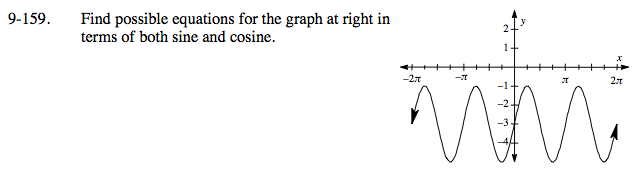### Home > PCT > Chapter Ch9 > Lesson 9.3.5 > Problem9-159

9-159.Sine Function
1. There is no horizontal shift.
2. It is shifted down 3.
3. The period is π. Find frequency: pb = 2π.
4. The amplitude is 2.

Cosine Function

$1. \ \ \text{The cosine is shifted to the right }\frac{\pi}{4}.$